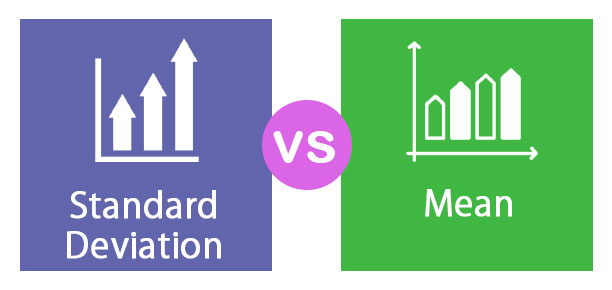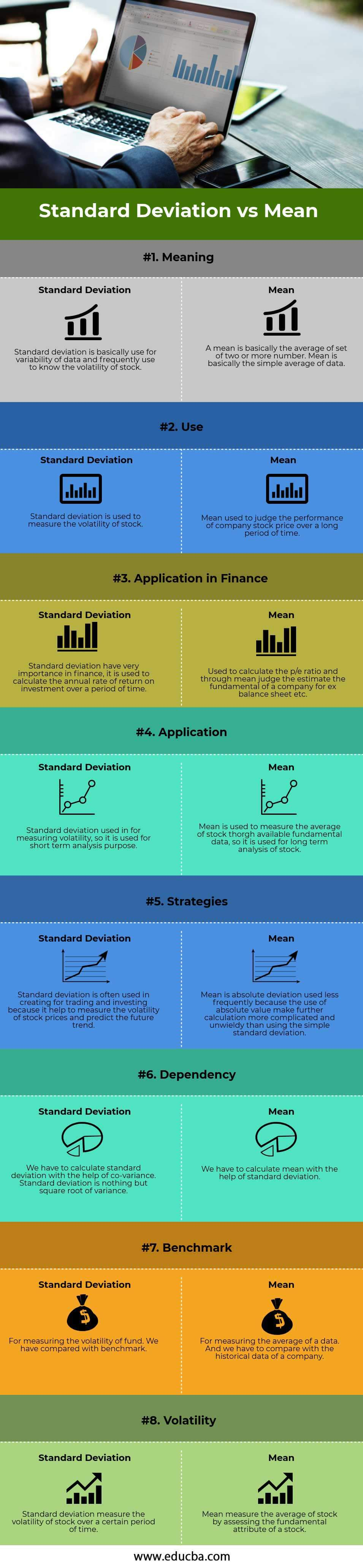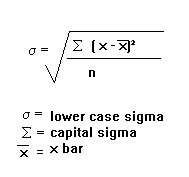# Standard Deviation vs Mean## Standard Deviation vs Mean

### Standard Deviation

Standard Deviation and Mean both the term used in statistics. Standard Deviation is statistics that basically measure the distance from the mean, and calculated as the square root of variance by determination between each data point relative to mean. Standard deviation plays a very important role in the world of finance. In finance standard deviation is a statistical measurement, when its applied to the annual rate of return of an investment. It sheds the volatility of historical volatility of that investment. The greater the standard deviation greater the volatility of an investment. Standard deviation is the best tool for measurement for volatility. Which help you to know the better and larger price range. Volatile stock has a very high standard deviation and blue chip stock have a very low standard deviation due to low volatility.

### Mean

Mean is a simple mathematical average of the set of two or more number. There are different types for calculation of mean, including the arithmetic mean method, which uses sums of all number in the series and geometric mean method. The simple method of mean is to make the total of all data and divide it by the number of data, then we reach to mean. Mean is nothing but the simple average of data. The mean is a statistical indicator which is used to judge the performance of a stock over a period of time through its earning over a period of time by assessing its fundamental such as P/E ratio, balance sheet, and the portfolio by estimating its average rate of return over a period of time.  So both Standard Deviation vs Mean term is used in statistics for calculation purpose.

Below is the top 8 difference between Standard Deviation vs Mean### Key Differences between Standard Deviation vs Mean

Let us discuss some of the major differences between Standard Deviation vs Mean

• Standard deviation is statistics that measure the dispersion of dataset relative to it is mean and its calculated as the square root of variance.it is calculated as the square root of variance by determining the variation between each data point relative to the mean.
• Mean is a mathematical average of the set of two or more number, the mean for the given number can be computed in more than one way.
• In fiancé standard deviation is used for calculation of an annual rate of return, whereas mean is calculated for the use of calculating the average with the help of historical data.
• Standard deviation used to measure the volatility of a stock, higher the standard deviation higher the volatility of a stock. Blue chip stock has low standard deviation so that have low volatility.
• It is the simplest form the mean is an average of all data point. Standard deviation is one of the key fundamental risk measures that analytics, portfolio managers, wealth management and financial planner used.
• Standard deviation is calculated based on the mean. In the stock market both the tool play a very important role in measuring the stock price and future performance of stock price and large price range.
• The formula of standard deviations:• The formula of mean -:

x¯¯¯=∑xN

### Standard Deviation vs Mean Comparison Table

Let’s look at the top 8 Comparison between Standard Deviation vs Mean

 The Basis of Comparision Between Standard Deviation vs Mean Standard Deviation Mean Meaning Standard deviation is basically used for the variability of data and frequently use to know the volatility of the stock. A mean is basically the average of a set of two or more number. Mean is basically the simple average of data. Use Standard deviation is used to measure the volatility of a stock. Mean used to judge the performance of company stock price over a long period of time. Application in finance Standard deviation has very importance in finance, it is used to calculate the annual rate of return on investment over a period of time. Used to calculate the p/e ratio and through mean judge the estimate the fundamental of a company forex balance sheet etc. Application Standard deviation used in for measuring volatility, so it is used for short term analysis purpose. Mean is used to measure the average of stock though available fundamental data, so it is used for long term analysis of a stock. Strategies Standard deviation is often used in creating for trading and investing because it helps to measure the volatility of stock prices and predict the future trend. Mean is absolute deviation used less frequently because the use of absolute value make the further calculation more complicated and unwieldy than using the sample standard deviation Dependency We have to calculate the standard deviation with the help of covariance. Standard deviation is nothing but the square root of the variance. We have to calculate the mean with the help of standard deviation. Benchmark For measuring the volatility of the fund. We have compared with the benchmark. For measuring the average of a data. And we have to compare with the historical data of a company. Volatility Standard deviation measures the volatility of the stock over a certain period of time. Mean measure the average of stock by assessing the fundamental attribute of a stock.

### Conclusion – Standard Deviation vs Mean

Standard deviation vs mean both the tool used for statistical valuation of stock price, both have their own importance in the field of finance. Big investor and companies apply these term for valuation of stock price and future prospectus. Standard deviation is the deviation from the mean, and a standard deviation is nothing but the square root of the variance. Mean is an average of all set of data available with an investor or company. Standard deviation used for measuring the volatility of a stock. So both Standard Deviation vs Mean plays a vital role in the field of finance. Standard deviation is easier to picture and apply. So both the tool used for strategies which can be used for application in trading and investment activity.

### Recommended Articles

This has a been a guide to the top difference between Standard Deviation vs Mean. Here we also discuss the standard Deviation vs Mean key differences with infographics and comparison table. You may also have a look at the following articles to learn more.

2. Creditor vs Debtor – Top Differences
3. Loan vs Lease – Which One Is Better
4. Expense vs Expenditure

The post Standard Deviation vs Mean appeared first on EDUCBA.

This post first appeared on Best Online Training & Video Courses | EduCBA, please read the originial post: here

# Share the post

Standard Deviation vs Mean

×